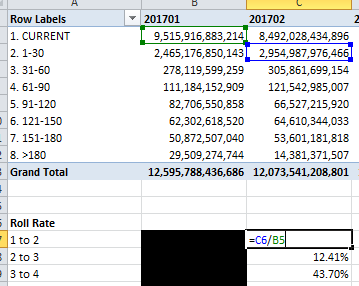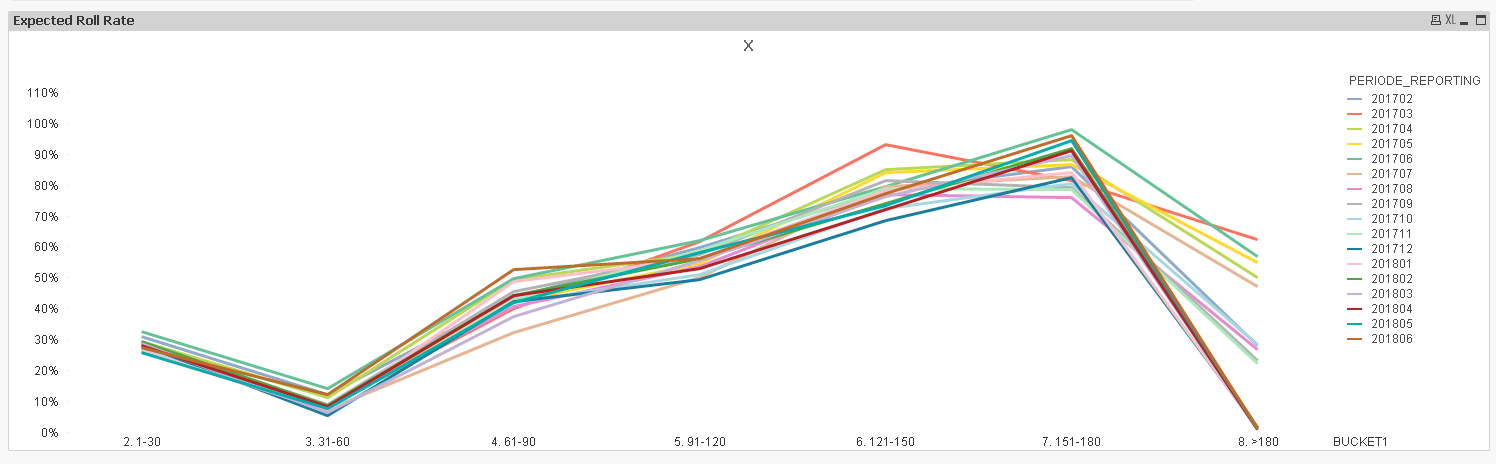# New to QlikView

Discussion board where members can get started with QlikView.

Highlighted
New Contributor

## Need Help to create roll rate line chart from above and before function

Hi All,

Basically, I want to create Roll rate/Flow rate/Delinquency rate from my data.

It was very easy on Excel (as attached sample), but on qlikview I was only able to show the flow rate number correctly using pivot chart

with expressions:

=sum(TotalOSPrincipal)/above(before(SUM(TotalOSPrincipal)))

when I convert the pivot chart, it show "no data to display", I think above and before function is not suitable for this case, any idea or alternative to get the line chart?

FYI, to get the flow rate number I use formula as below :then easily create line chart based on roll rate number.

1 Solution

Accepted SolutionsMVP

## Re: Need Help to create roll rate line chart from above and before function

Try this expression

=Sum(TotalOSPrincipal)/Aggr(Above(Aggr(Above(Sum(TotalOSPrincipal)), BUCKET1, PERIODE_REPORTING)), PERIODE_REPORTING, BUCKET1)4 RepliesMVP

## Re: Need Help to create roll rate line chart from above and before function

Try this expression

=Sum(TotalOSPrincipal)/Aggr(Above(Aggr(Above(Sum(TotalOSPrincipal)), BUCKET1, PERIODE_REPORTING)), PERIODE_REPORTING, BUCKET1)MVP

## Re: Need Help to create roll rate line chart from above and before function

Or this

=Sum(TotalOSPrincipal)/Only({<BUCKET1, PERIODE_REPORTING>}Aggr(Above(Only({<BUCKET1, PERIODE_REPORTING>}Aggr(Above(SUM({<BUCKET1, PERIODE_REPORTING>}TotalOSPrincipal)), BUCKET1, PERIODE_REPORTING))), PERIODE_REPORTING, BUCKET1))

New Contributor

## Re: Need Help to create roll rate line chart from above and before function

Hi Sunny,

Thank You very much, it's work flawlessly!

I took so many time to resolve this using set analysis, but suddenly you solved this using Aggregation and above function.

I should learn again about those function, to know better when to use it.

Have a nice day...MVP

## Re: Need Help to create roll rate line chart from above and before function

Just remember that set analysis is evaluated once per chart, so it can't be used for scenarios like these where you expecting a different value from set analysis every dimension. In these scenarios, Inter-Record Functions come in handy